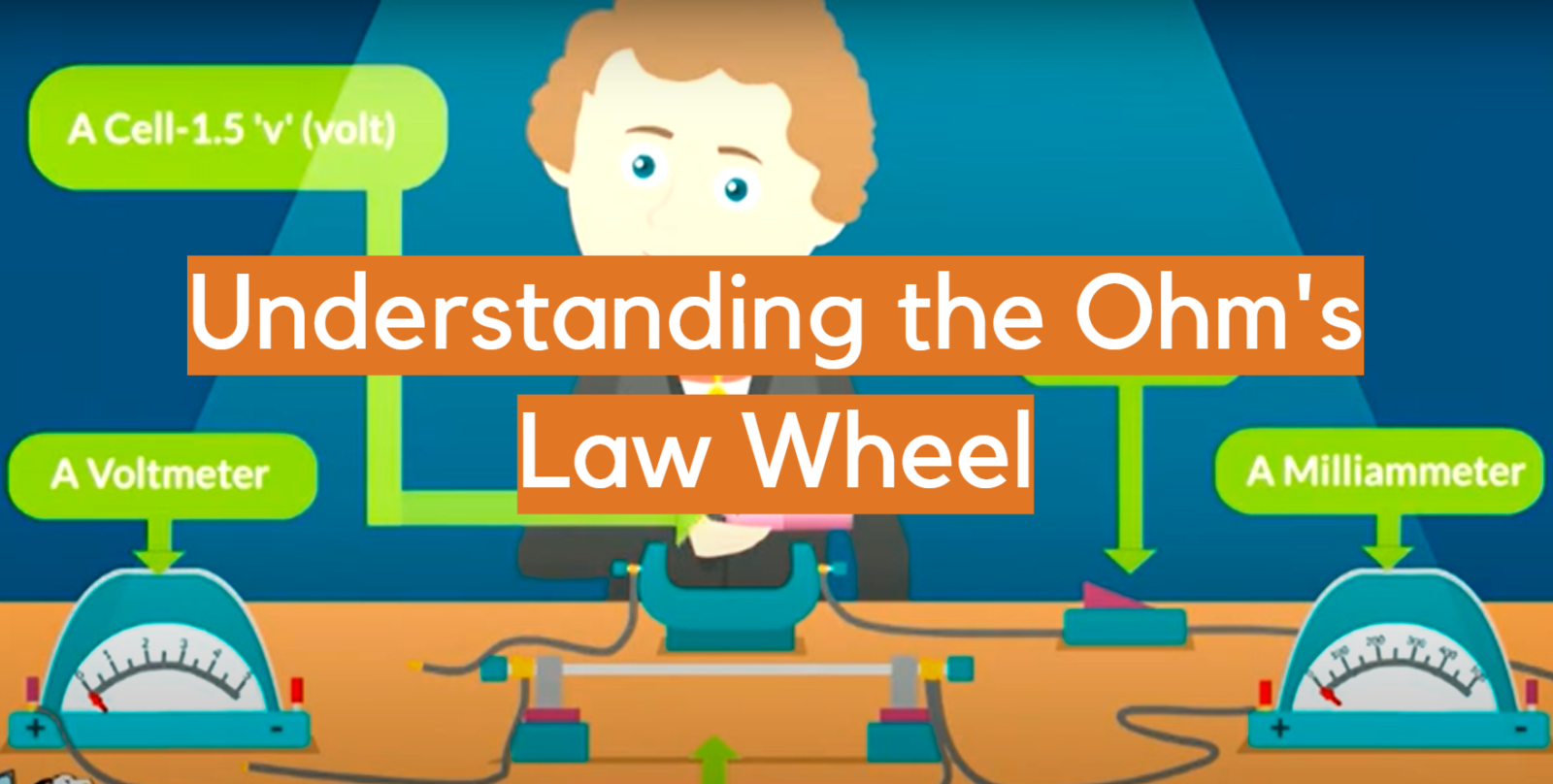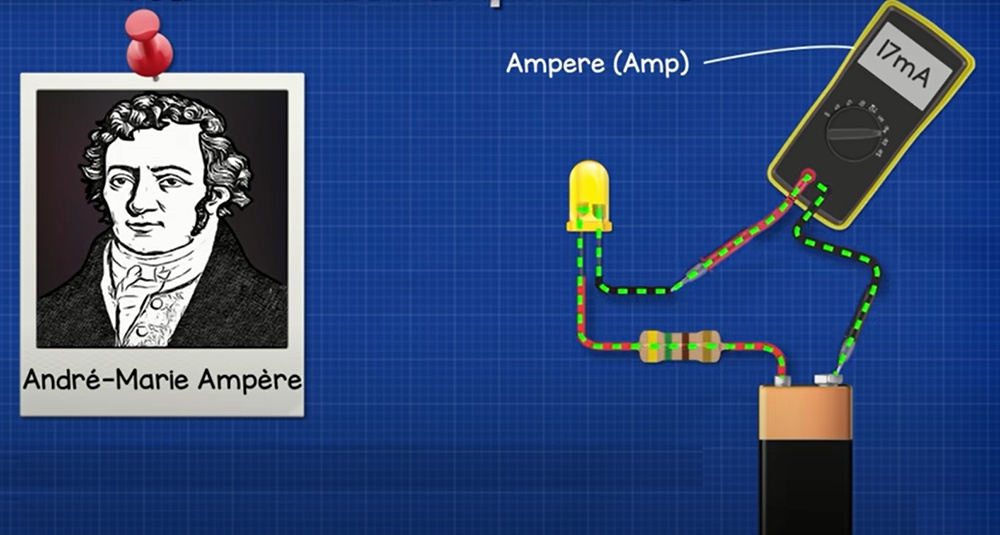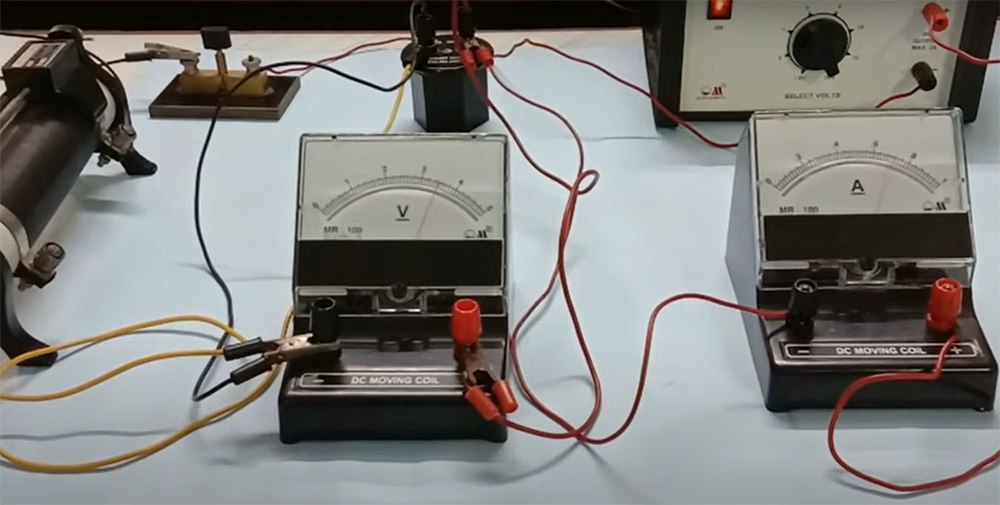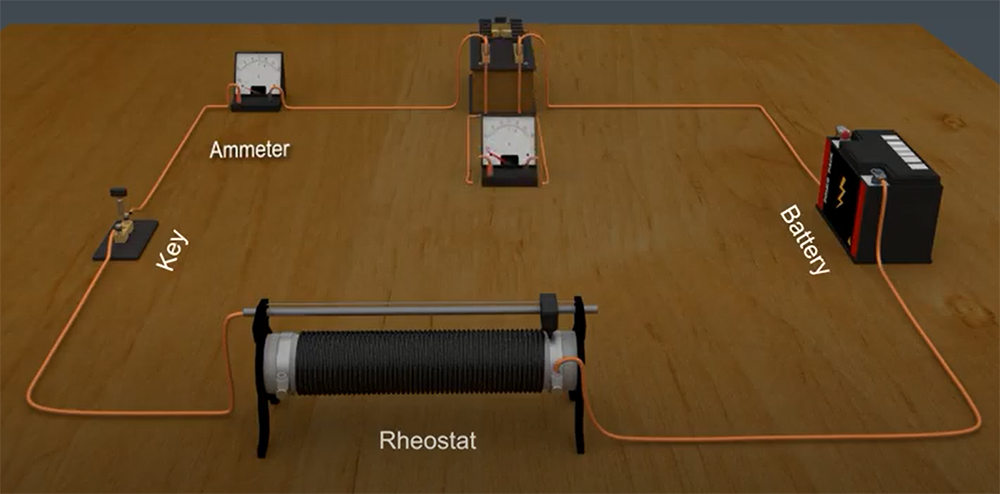# Understanding the Ohm’s Law WheelIf you’re looking for a comprehensive guide to the Ohm’s Law Wheel, look no further! In this blog post, we will discuss everything you need to know about electricity. We’ll start by talking about the different parts of Ohm’s Law Wheel and what they mean. Then, we’ll go over some common electrical terms and concepts. Finally, we’ll talk about how to use Ohm’s Law Wheel to solve problems. Are you ready to learn about electricity? Let’s get started!

## What is Ohm’s Law?

Ohm’s Law is the most important equation in all of the electricity, and it’s vital to understanding how electrical circuits work .

If you double the voltage, you will also double the current.

It is also worth noting that Ohm’s Law equation only applies to DC circuits. AC circuits are a bit more complicated, but they can be simplified using the power factor.## Who Developed Ohm’s Law?

Physicist Georg Simon Ohm was a German who discovered the relationship between resistance, current, and voltage. This discovery became known as Ohm’s Law. In 1827, he published his findings.

Ohm’s law is represented by the equation: V = IR

This equation is known as Ohm’s law formula.

## How to Use Ohm’s Law

Now that we’ve gone over what each symbol in the Ohm’s Law equation represents, it’s time to put this knowledge to use and learn how to calculate electrical values .

The first thing you need to do is identify which two of the three values you know. Once you have done that, you can solve for the unknown value using the following equation:

For example, you want to calculate V across a resistor with a resistance of 12 Ω. In this case, you would use the equation V = IR. Plugging in the values, you get:

V = 12Ω * I

Now, all you have to do is solve for I to get the value of current. In this case, I = V/R, so:

I = 12/12

From this, we can see that the voltage across the resistor is equal to the amount of current flowing through it.

Now you can plug in different values to solve for the unknown quantity.

Just remember, this equation is only valid when resistance is constant. If the resistance changes, so will the current and voltage.

## What is Ohm’s Law Wheel?

The Ohm’s Law Wheel is a very useful tool for understanding electricity and how it works. It is a visual guide that illustrates the relationship between resistance, current, and voltage.

The wheel is divided into four quadrants. Each of them represents a different value of either V, I, or R. The values of the quadrants are:

• Voltage: The potential difference between two points. Measured in volts.
• Current: The rate at which charge flows through a conductor. Measured in amperes.
• Resistance: The opposition to the flow of current. Measured in ohms.
• Power (P): The rate at which work is done. Measured in watts.To use the Ohm’s Law Wheel, simply find the quadrant that corresponds to the value you are trying to find.

Also, the Ohm’s Law Wheel can be used to find the value of any two variables if the third variable is known. Let’s say you know the values of resistance and voltage, you can find the value of current by finding the quadrant that contains those values.

If you are ever unsure about how electricity works, be sure to consult the Ohm’s Law Wheel.

It is important to note that the Ohm’s Law Wheel is not a substitute for actual knowledge of electricity. It is merely a tool to help you understand the concepts. If you want to learn more about electricity, be sure to consult other sources as well.

## When to Use Ohm’s Law Wheel

Now that we’ve gone over the basics of Ohm’s law wheel, you might be wondering when it would come in handy. The truth is, this tool can be used in a variety of situations!

Here are just a few examples:

• You’re working with a new electrical device and want to understand its capabilities – Ohm’s law will tell you how much current the device can handle.
• You want to know how to properly wire an electrical circuit – by using the wheel, you can determine the correct gauge of wire to use.
• You’re troubleshooting an electrical issue – Ohm’s law can help you pinpoint the problem area in a circuit.
• You need to calculate the power dissipation of a resistor – the power dissipation formula is included on Ohm’s law wheel!In general, Ohm’s law wheel is a great tool to have on hand whenever you’re working with electricity. It’s easy to use and can be a real lifesaver in a pinch.

As you can see, Ohm’s law wheel is a versatile tool that can be used in a variety of situations. There are countless other uses for Ohm’s law wheel – these are just a few examples.

So whether you’re a beginner just learning the basics of electricity or a seasoned professional, this guide will come in handy. So, the next time you’re working with electricity, be sure to pull out your trusty Ohm’s law wheel!

## Ohm’s Law Example Problems

Now that you know how to use Ohm’s Law Wheel, let’s go over some example problems. These will help you see how the wheel can be used in practice to calculate different values related to electric current, voltage, and resistance.

Example Problem #01:

You have a light bulb with a resistance of 50 ohms. You know that the voltage in your home’s electrical system is 120 volts. What is the current flowing through the light bulb?

• To solve this problem, simply find the point on the wheel where “V” and “R” intersect. So that point is at “I” (current). And you have the current flowing through the light bulb is 0.24 amps.

Example Problem #02:

You have a toaster with a resistance of 30 ohms. You know that the current flowing through the toaster is 0.50 amps. What is the voltage across the toaster?

• To find the solution, look at the point on the wheel where “I” (current) and “R” (resistance) intersect. In this way, that point is at “V” (voltage). Now you know that the voltage across the toaster is 15 volts.

Example Problem #03:

You have a car battery with a voltage of 12 volts. You know that the current flowing through the car battery is 25 amps. What is the resistance of the car battery?

• For solving the problem, take the point on the wheel where “I” and “V” intersect. In this case, that point is at resistance. So the resistance of the car battery is 0.48 ohms.

Example Problem #04:You want to build a circuit with a voltage of 12 volts and a current of 0.50 amps. What should the resistance of the circuit be?

• To solve this problem, find the point on the wheel where current and voltage intersect. Now that point is at “R”. Here we get that the resistance of the circuit should be 24 ohms.

As you can see, using Ohm’s Law Wheel is a quick and easy way to solve problems involving electric current, voltage, and resistance. In just a few seconds, you can figure out values that would otherwise take much longer to calculate.

## Principles of Ohm’s Law

There is two primary principles of Ohm’s Law: proportional and inverse proportionality.

• Proportional: This is when the voltage and current are equal. In this instance, the resistance remains the same.
• Inverse Proportionality: This is when the voltage and current are inversely proportional to each other. In this principle, the resistance changes.

These principles are what Ohm’s Law Wheel is based on.

## Ohm’s Law Wheel: A Comparison of Key Indicators

To fully grasp its importance, it is essential to understand the various indicators involved in its use. This table provides a comparison of the key indicators related to the Ohm’s Law Wheel, including their definitions, formulas, and units of measurement. By understanding these indicators, individuals can gain a deeper understanding of how to apply the Ohm’s Law Wheel in solving complex problems in electrical circuits.

Indicator Definition Formula Unit
Voltage The electrical potential difference between two points in a circuit V = IR Volts (V)
Current The flow of electric charge through a circuit I = V/R Amps (A)
Resistance The opposition to the flow of electric charge R = V/I Ohms (Ω)
Power The rate at which energy is transferred in a circuit P = IV Watts (W)
Ohm’s Law Wheel A tool used to solve for voltage, current, resistance, and power in a circuit N/A N/A

Note that the Ohm’s Law Wheel does not have a specific formula or unit, as it is a visual aid to help solve for voltage, current, resistance, and power using the three formulas listed above.

## FAQ

### Why do we use Ohm’s law?

Ohm’s law is used to describe the relationship between V, I, and R in an electrical circuit. By using this law, we can determine the amount of current flowing through a circuit given the voltage and resistance. Additionally, we can calculate the V drop across a resistor given the current and resistance. Finally, we can determine the resistance of a material given the voltage and current.

### How is Ohm’s law used in everyday life?

Ohm’s law is used in a variety of ways in our everyday lives. For example, when we charge our phones overnight, we are using Ohm’s law to ensure that the correct amount of current flows through the charging cord so that our phones do not overcharge. Additionally, when we adjust the volume on our stereo systems, we are using Ohm’s law to calculate the resistance in the circuit so that we can control the amount of current flowing through the speakers.

### Why is I current in electronics?

The letter “I” is used to represent current in electronics because it is the symbol for intensity. In an electrical circuit, a current is the measure of the flow of charge through a material. The SI unit for measuring current is the ampere (A).

### What is the difference between AC and DC current?

AC and DC current are two types of current that flow through electrical circuits.

• AC flows in one direction, then reverses direction periodically. The frequency of this reversal is measured in hertz (Hz).
• DC flows in one direction only.

AC is the type of current that is used in our homes, while DC is the type of current that is used in batteries.

### Why is the Ohm’s law wheel used in electrical engineering?

The Ohm’s law wheel is a graphical representation of the relationships between voltage, current, and resistance. It is a useful tool for understanding how these quantities interact with each other in electrical circuits.

The Ohm’s law wheel can be used to determine the values of unknown quantities in a circuit, as well as to analyze the behavior of circuits under various conditions. It is also a valuable teaching tool, as it can help students visualize the relationships between these important concepts.

### How can the Ohm’s law wheel be used to solve problems?

The Ohm’s law wheel can be used to solve problems by understanding the relationships between voltage, current, and resistance. By using the wheel, you can determine the values of any two variables if the third is known. For example, if you know the voltage and resistance in a circuit, you can use the wheel to find the value of the current.

Another way to use the Ohm’s law wheel is to find out what happens when one of the variables in a circuit is changed. For example, if you increase the resistance in a circuit, how will that affect the current? You can use the Ohm’s law wheel to find out.

Finally, you can use the Ohm’s law wheel to troubleshoot problems in a circuit. For example, if you know the voltage and current in a circuit, but the resistance is unknown, you can use the wheel to find out what the resistance might be.

### What are some of the limitations of the Ohm’s law wheel?

The Ohm’s law wheel is a great tool to help you visualize the relationships between voltage, current, and resistance. However, there are some limitations to using this tool. One of the biggest limitations is that it only applies to linear circuits. This means that if you have a circuit with multiple resistors or other non-linear components, the Ohm’s law wheel will not be accurate.

### What are some of the benefits of using the Ohm’s law wheel?

The Ohm’s law wheel is a great tool for understanding electrical circuits. It can help you determine the resistance of a circuit, the voltage drop across a resistor, and the current through a circuit. Additionally, the Ohm’s law wheel can be used to find the power dissipated in a circuit.

Another benefit of using the Ohm’s law wheel is that it can help you solve complex problems. For example, if you know the voltage across a resistor and the current flowing through it, you can use the Ohm’s law wheel to find the resistance of the resistor.

Finally, the Ohm’s law wheel is a handy tool to have around when working with electrical circuits. It can help you troubleshoot problems and make calculations quickly and easily.

### Are there any drawbacks to using the Ohm’s law wheel?

The main drawback of using the Ohm’s law wheel is that it can be confusing to look at and understand. The wheel is divided into different sections, each with its own symbol and value. If you don’t know what you’re looking at, it can be difficult to make sense of it all.

Ohm’s law also cannot be applied to non-linear systems. This includes systems where the resistance changes with voltage or current, such as in a transistor. In these cases, other laws must be used to determine the relationship between voltage, current, and resistance.

### How do you memorize the Ohm’s law wheel?

One common mistake that students make when trying to memorize the Ohm’s law wheel is trying to memorize all of the information at once. This is often overwhelming and can lead to frustration. Instead, it is important to focus on one small section at a time. For instance, start by memorizing the formula for Ohm’s law (V = IR). Then, once you have mastered that, move on to memorizing the definitions of voltage, current, and resistance. Once you have mastered those basics, you can then move on to more complicated concepts such as parallel and series circuits. Whichever method you choose, it is important to practice regularly so that you can recall the information when you need it.

### What does P stand for in Ohm’s law?

P stands for power and is measured in watts. Power is the rate at which work is done or energy is used. In Ohm’s law, P=IV, so power is equal to current times voltage.

The standard unit of measurement for electrical power is the watt (W). One watt is equal to one joule per second (J/s). Electrical power can be calculated using either the voltage and current values, or the resistance value with either voltage or current.

### Is Ohm’s law always true?

No, Ohm’s law is not always true. It is only true when the resistance in a circuit remains constant. If the resistance changes, then Ohm’s law no longer applies.

However, there are certain conditions where Ohm’s law can be approximated to be true. For example, if the voltage and current in a circuit are small and the temperature is constant, then Ohm’s law will hold true.

In such cases, Ohm’s law is a 100% accurate model of how current flows through a resistor.

## Final Thoughts

Whether you’re a student, a professional, or just someone who’s interested in electricity, understanding the Ohm’s Law Wheel is essential. With this guide, you should now have a clear understanding of how the wheel works and what it means.

Remember, the Ohm’s Law Wheel is a powerful tool that can be used to solve a variety of problems. By understanding the relationships between V, I, and R, you can troubleshoot electrical issues, design circuits, and much more.

If you’re ever stuck on a problem or don’t know where to start, refer back to this guide. With practice, you’ll be able to use Ohm’s Law Wheel with ease. Thanks for reading!

I hope this guide was helpful in understanding Ohm’s Law Wheel. If you have any questions, feel free to leave a comment below and I’ll do my best to answer them. Thanks for reading!